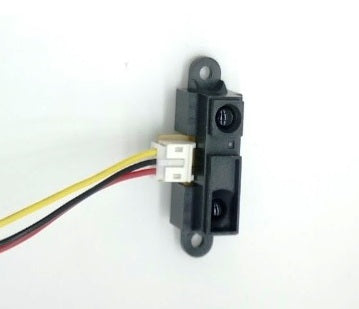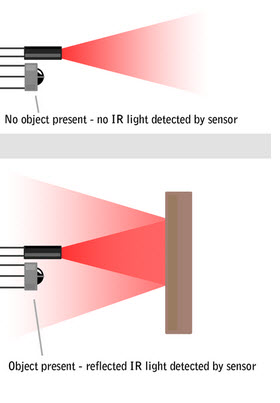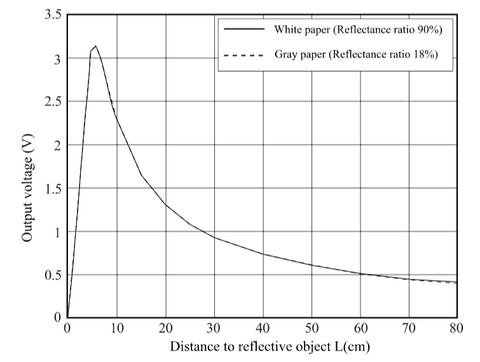# Analog IR Distance Sensor

In this tutorial, we will learn how to use the Analog IR Distance Sensor with a simple example. We are using the GP2Y0A21YK0F optical sensor which is extremely useful in detecting very close objects (10 cm to 80 cm).

Components Required:

Software Required:How the sensor works?

The sensor contains an IR LED and a photodiode sensor. The LED continuously produces light of a specific wavelength. Whenever an object is present in front of the light, the light is reflected back. The photodiode is sensitive to this wavelength of light and produces current depending on the intensity of light received after reflection.

If the object is far from the sensor, less amount of light is reflected back and the photodiode produces less current.Step 1 : Connect sensor with Arduino Uno

 Analog IR Sensor Arduino UNO VCC ( red wire) 5V GND (black wire) GND Signal (yellow wire) A0Step 2 : Upload and Run the Code

Connect the Arduino to the computer using the USB Cable.
Open Arduino IDE software
Select the appropriate board under Tools > Board
Select the appropriate Serial Port under Tools > Port

Here is an example code that you can upload. It will print the reading from IR Analog Sensor on the serial monitor.You can calculate the distance by multiplying the sensor reading by (5/1024). We multiply by this value to convert analogRead() value to voltage. Now use this voltage to find the distance using the following graph:Datasheet for GP2Y0A21YK0F

Author: Nakul Garg